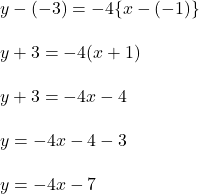## 6.Write the equation of the line in slope-intercept form that has a slope of -4 and passes through the point (-1, -3).

Question

6.Write the equation of the line in slope-intercept form that has a slope of -4 and passes through the point (-1, -3).

in progress 0
3 weeks 2021-08-23T23:25:53+00:00 1 Answers 0 views 0